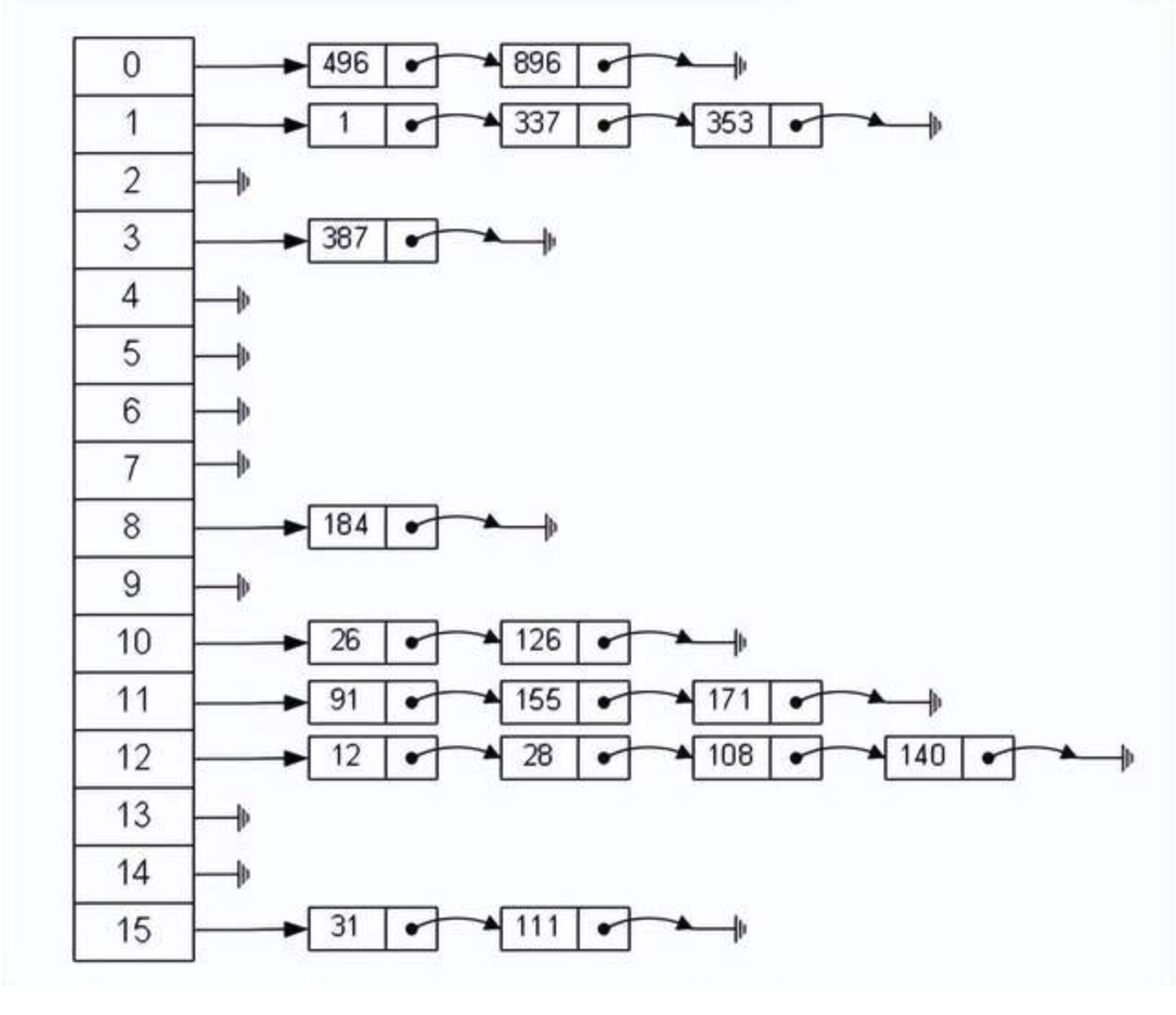## 一、HashMap概述

​ HashMap基于哈希表的 Map 接口的实现。此实现提供所有可选的映射操作，并允许使用 null 值和 null 键。（除了不同步和允许使用 null 之外，HashMap 类与 Hashtable 大致相同。）此类不保证映射的顺序，特别是它不保证该顺序恒久不变。

​ 值得注意的是HashMap不是线程安全的，如果想要线程安全的HashMap，可以通过Collections类的静态方法synchronizedMap获得线程安全的HashMap。

``````Map map = Collections.synchronizedMap(new HashMap());

Map<Object, Object> map = new ConcurrentHashMap<>();	//效果同上
``````

## 二、HashMap的数据结构

​ HashMap的底层主要是基于数组和链表来实现的，它之所以有相当快的查询速度主要是因为它是通过计算散列码来决定存储的位置。HashMap中主要是通过key的hashCode来计算hash值的，只要hashCode相同，计算出来的hash值就一样。如果存储的对象对多了，就有可能不同的对象所算出来的hash值是相同的，这就出现了所谓的hash冲突。学过数据结构的同学都知道，解决hash冲突的方法有很多，HashMap底层是通过链表来解决hash冲突的。HashMap其实也是一个线性的数组实现的,所以可以理解为其存储数据的容器就是一个线性数组。这可能让我们很不解，一个线性的数组怎么实现按键值对来存取数据呢？这里HashMap有做一些处理。

``````/** Entry是单向链表。
* 它是 “HashMap链式存储法”对应的链表。
*它实现了Map.Entry 接口，即实现getKey(), getValue(), setValue(V value), equals(Object o), hashCode()这些函数
**/
static class Entry<K,V> implements Map.Entry<K,V> {
final K key;
V value;
// 指向下一个节点
Entry<K,V> next;
final int hash;

// 构造函数。
// 输入参数包括"哈希值(h)", "键(k)", "值(v)", "下一节点(n)"
Entry(int h, K k, V v, Entry<K,V> n) {
value = v;
next = n;
key = k;
hash = h;
}

public final K getKey() {
return key;
}

public final V getValue() {
return value;
}

public final V setValue(V newValue) {
V oldValue = value;
value = newValue;
return oldValue;
}

// 判断两个Entry是否相等
// 若两个Entry的“key”和“value”都相等，则返回true。
// 否则，返回false
public final boolean equals(Object o) {
if (!(o instanceof Map.Entry))
return false;
Map.Entry e = (Map.Entry)o;
Object k1 = getKey();
Object k2 = e.getKey();
if (k1 == k2 || (k1 != null && k1.equals(k2))) {
Object v1 = getValue();
Object v2 = e.getValue();
if (v1 == v2 || (v1 != null && v1.equals(v2)))
return true;
}
return false;
}

// 实现hashCode()
public final int hashCode() {
return (key==null   ? 0 : key.hashCode()) ^
(value==null ? 0 : value.hashCode());
}

public final String toString() {
return getKey() + "=" + getValue();
}

// 当向HashMap中添加元素时，绘调用recordAccess()。
// 这里不做任何处理
void recordAccess(HashMap<K,V> m) {
}

// 当从HashMap中删除元素时，绘调用recordRemoval()。
// 这里不做任何处理
void recordRemoval(HashMap<K,V> m) {
}
}
``````

HashMap其实就是一个Entry数组，Entry对象中包含了键和值，其中next也是一个Entry对象，它就是用来处理hash冲突的，形成一个链表。

## 三、HashMap源码分析

### 1、关键属性

`````` transient Entry[] table;//存储元素的实体数组

transient int size;//存放元素的个数

int threshold; //临界值   当实际大小超过临界值时，会进行扩容threshold = 加载因子*容量

transient int modCount;//被修改的次数
``````

### 2、构造方法

``````public HashMap(int initialCapacity, float loadFactor) {
//确保数字合法
if (initialCapacity < 0)
throw new IllegalArgumentException("Illegal initial capacity: " +
initialCapacity);
if (initialCapacity > MAXIMUM_CAPACITY)
initialCapacity = MAXIMUM_CAPACITY;
throw new IllegalArgumentException("Illegal load factor: " +

// Find a power of 2 >= initialCapacity
int capacity = 1;   //初始容量
while (capacity < initialCapacity)   //确保容量为2的n次幂，使capacity为大于initialCapacity的最小的2的n次幂
capacity <<= 1;

table = new Entry[capacity];
init();
}

public HashMap(int initialCapacity) {
}

public HashMap() {
table = new Entry[DEFAULT_INITIAL_CAPACITY];
init();
}
``````

### 3、存储数据

``````public V put(K key, V value) {
// 若“key为null”，则将该键值对添加到table中。
if (key == null)
return putForNullKey(value);
// 若“key不为null”，则计算该key的哈希值，然后将其添加到该哈希值对应的链表中。
int hash = hash(key.hashCode());
//搜索指定hash值在对应table中的索引
int i = indexFor(hash, table.length);
// 循环遍历Entry数组,若“该key”对应的键值对已经存在，则用新的value取代旧的value。然后退出！
for (Entry<K,V> e = table[i]; e != null; e = e.next) {
Object k;
if (e.hash == hash && ((k = e.key) == key || key.equals(k))) { //如果key相同则覆盖并返回旧值
V oldValue = e.value;
e.value = value;
e.recordAccess(this);
return oldValue;
}
}
//修改次数+1
modCount++;
//将key-value添加到table[i]处
return null;
}
``````

`````` private V putForNullKey(V value) {
for (Entry<K,V> e = table; e != null; e = e.next) {
if (e.key == null) {   //如果有key为null的对象存在，则覆盖掉
V oldValue = e.value;
e.value = value;
e.recordAccess(this);
return oldValue;
}
}
modCount++;
return null;
}
``````

``````//计算hash值的方法 通过键的hashCode来计算
static int hash(int h) {
// This function ensures that hashCodes that differ only by
// constant multiples at each bit position have a bounded
// number of collisions (approximately 8 at default load factor).
h ^= (h >>> 20) ^ (h >>> 12);
return h ^ (h >>> 7) ^ (h >>> 4);
}
``````

`````` static int indexFor(int h, int length) { //根据hash值和数组长度算出索引值
return h & (length-1);  //这里不能随便算取，用hash&(length-1)是有原因的，这样可以确保算出来的索引是在数组大小范围内，不会超出
}
``````

``````         h & (table.length-1)                     hash                             table.length-1
8 & (15-1)：                                 1000                   &              1110                   =                1000
9 & (15-1)：                                 1001                   &              1110                   =                1000
-----------------------------------------------------------------------------------------------------------------------
8 & (16-1)：                                 1000                   &              1111                   =                1000
9 & (16-1)：                                 1001                   &              1111                   =                1001
``````

• 如果两个 Entry 的 key 的 hashCode() 返回值相同，那它们的存储位置相同。

• 如果这两个 Entry 的 key 通过 equals 比较返回 true，新添加 Entry 的 value 将覆盖集合中原有 Entry 的 value，但key不会覆盖。

• 如果这两个 Entry 的 key 通过 equals 比较返回 false，新添加的 Entry 将与集合中原有 Entry 形成 Entry 链，而且新添加的 Entry 位于 Entry 链的头部——

`````` void addEntry(int hash, K key, V value, int bucketIndex) {
Entry<K,V> e = table[bucketIndex]; //如果要加入的位置有值，将该位置原先的值设置为新entry的next,也就是新entry链表的下一个节点
table[bucketIndex] = new Entry<>(hash, key, value, e);
if (size++ >= threshold) //如果大于临界值就扩容
resize(2 * table.length); //以2的倍数扩容
}
``````

### 4、调整大小

resize()方法如下：

`````` void resize(int newCapacity) {
Entry[] oldTable = table;
int oldCapacity = oldTable.length;
if (oldCapacity == MAXIMUM_CAPACITY) {
threshold = Integer.MAX_VALUE;
return;
}

Entry[] newTable = new Entry[newCapacity];
transfer(newTable);//用来将原先table的元素全部移到newTable里面
table = newTable;  //再将newTable赋值给table
}
``````

### 5、数据读取

``````public V get(Object key) {
if (key == null)
return getForNullKey();
int hash = hash(key.hashCode());
for (Entry<K,V> e = table[indexFor(hash, table.length)];
e != null;
e = e.next) {
Object k;
if (e.hash == hash && ((k = e.key) == key || key.equals(k)))
return e.value;
}
return null;
}
``````

### 6、HashMap的性能参数

HashMap 包含如下几个构造器：

HashMap()：构建一个初始容量为 16，负载因子为 0.75 的 HashMap。

HashMap(int initialCapacity)：构建一个初始容量为 initialCapacity，负载因子为 0.75 的 HashMap。

``````threshold = (int)(capacity * loadFactor);